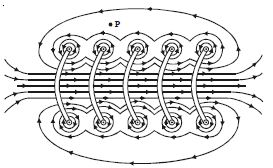# Define and Describe on Solenoid

A long closely wound helical coil is called a solenoid. Figure shows a section of stretched out solenoid. The magnetic field due to the solenoid is the vector sum of the magnetic fields due to current through individual turns of the solenoid.

The magnetic fields associated with each single turn are almost concentric circles and hence tend to cancel between the turns. At the interior midpoint, the field is strong and along the axis of the solenoid (i.e) the field is parallel to the axis.Fig: Magnetic field due to a current carrying solenoid.

For a point such as P, the field due to the upper part of the solenoid turns tend to cancel the field due to the lower part of the solenoid turns, acting in opposite directions. Hence the field outside the solenoid is nearly zero. The direction of the magnetic field due to circular closed loops (solenoid) is given by right hand palm-rule.